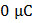# A thermister is dipped in a bath whose temperature is to be measured. When the temperature increase the current also increase, because of decreases in a) Capacitance b) Reactance c) Resistance d) Resistivity

## Question ID - 151343 :- A thermister is dipped in a bath whose temperature is to be measured. When the temperature increase the current also increase, because of decreases in a) Capacitance b) Reactance c) Resistance d) Resistivity

3537

 (d) The temperature coefficient of resistivity for thermister is negative. Therefore by increase in temperature the resistivity of the thermister decreases.

Next Question :

In the given circuit diagram the current through the battery and the charge on the capacitor respectively in steady state area)andb)andc)andd)and